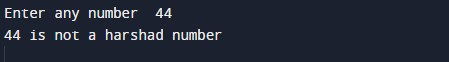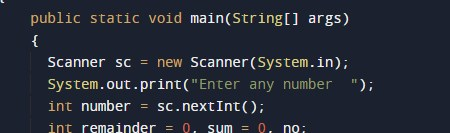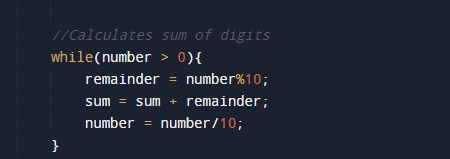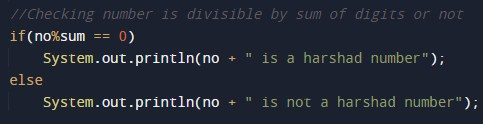# Program to check Harshad Number In Java

In this tutorial you will learn about the Program to check Harshad Number In Java and its application with practical example.

In this tutorial, we will learn to create a Java Program to check Harshad  Numbers in Java using Java programming.

## Prerequisites

Before starting with this tutorial we assume that you are best aware of the following Java programming topics:

• Java Operators.
• Basic Input and Output function in Java.
• Class and Object in Java.
• Basic Java programming.
• If-else statements in Java.
• For loop in Java.

Harshad number is a number that if it is divisible by the sum of its digit.

For example:=> 40, Sum of digit is (4 +0 = 4). So 40 is divisible by 4. So, 40 is a Harshad number.

## Program to check Harshad Number in Java

In this program we would find given number is a Harshad  number or not .first of we would take a value from user and find the Harshad  number.let’s  have a look at the code.

## OutputIn the above program, we have first declared and initialized a set variables required in the program.

• no and number= it will hold entered number.
• remainder = it will hold remainder of values.
• sum = it will hold sum of digit

After that we take a number from user and find given number is a Harshad number or not### Algorithm

1. First of all we take and initialize variable number.
2. Duplicate the number in variable no.
3. check Condition while number is greater than 0, then find remainder of number by dividing number with 10.1. Add remainder to a variable sum=>(sum = sum + remainder).
2. Divide number by 10.
3. Repeat the steps 3 to 5,If no divide sum completely, then number is a Harshad number. Else, given number is not a Harshad number.

In this tutorial we have learn about the Program to check Harshad Number In Java and its application with practical example. I hope you will like this tutorial.